Find Paper, Faster
Example：10.1021/acsami.1c06204 or Chem. Rev., 2007, 107, 2411-2502
UNEXPECTED AVERAGE VALUES OF GENERALIZED VON MANGOLDT FUNCTIONS IN RESIDUE CLASSES
Journal of the Australian Mathematical Society  (IF0.8),  Pub Date : 2020-07-17, DOI: 10.1017/s1446788719000715
NICOLAS ROBLES, ARINDAM ROY

In order to study integers with few prime factors, the average of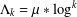$\unicode[STIX]{x1D6EC}_{k}=\unicode[STIX]{x1D707}\ast \log ^{k}$ has been a central object of research. One of the more important cases,$k=2$ , was considered by Selberg [‘An elementary proof of the prime-number theorem’, Ann. of Math. (2) 50 (1949), 305–313]. For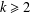$k\geq 2$ , it was studied by Bombieri [‘The asymptotic sieve’, Rend. Accad. Naz. XL (5) 1(2) (1975/76), 243–269; (1977)] and later by Friedlander and Iwaniec [‘On Bombieri’s asymptotic sieve’, Ann. Sc. Norm. Super. Pisa Cl. Sci. (4) 5(4) (1978), 719–756], as an application of the asymptotic sieve.

Let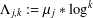$\unicode[STIX]{x1D6EC}_{j,k}:=\unicode[STIX]{x1D707}_{j}\ast \log ^{k}$ , where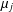$\unicode[STIX]{x1D707}_{j}$ denotes the Liouville function for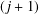$(j+1)$ -free integers, and$0$ otherwise. In this paper we evaluate the average value of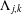$\unicode[STIX]{x1D6EC}_{j,k}$ in a residue class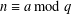$n\equiv a\text{ mod }q$ ,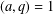$(a,q)=1$ , uniformly on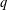$q$ . When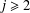$j\geq 2$ , we find that the average value in a residue class differs by a constant factor from the expected value. Moreover, an explicit formula of Weil type for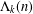$\unicode[STIX]{x1D6EC}_{k}(n)$ involving the zeros of the Riemann zeta function is derived for an arbitrary compactly supported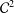${\mathcal{C}}^{2}$ function.